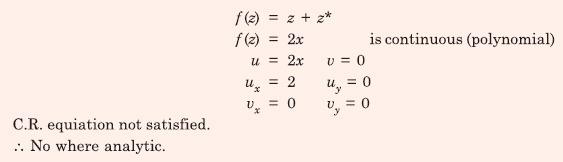# EE GATE 2016 SET 2 - Online Test

Q1. The Boolean expression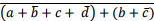simplifies to
Explaination / Solution:Q2. Pick the odd one out in the following: 13, 23, 33, 43, 53
Explaination / Solution: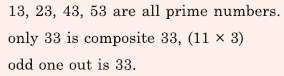Q3. If |9y−6| = 3, then y2 −4y/3 is ________ .
Explaination / Solution: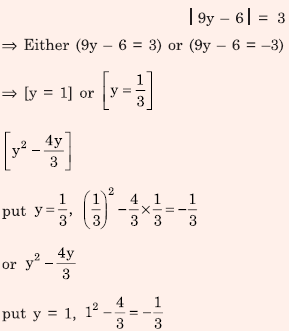Q4. A power system with two generators is shown in the figure below. The system (generators, buses and transmission lines) is protected by six overcurrent relays R1 to R6. Assuming a mix of directional and nondirectional relays at appropriate locations, the remote backup relays for R4 are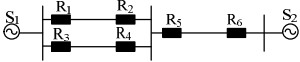Explaination / Solution:
No Explaination.

Q5. A power system has 100 buses including 10 generator buses. For the load flow analysis using Newton-Raphson method in polar coordinates, the size of the Jacobian is
Explaination / Solution:Q6. The direction of rotation of a single-phase capacitor run induction motor is reversed by
Explaination / Solution:
No Explaination.

Q7. Consider a causal LTI system characterized by differential equation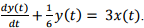The response of the system to the inputwhere u(t) denotes the unit step function, is
Explaination / Solution: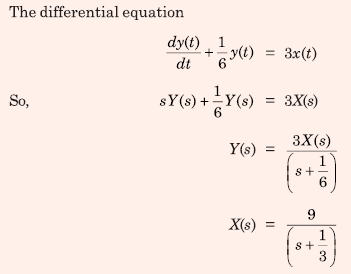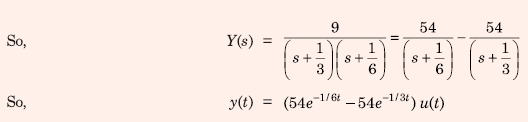Q8. The inductance and capacitance of a 400 kV, three-phase, 50 Hz lossless transmission line are 1.6 mH/km/phase and 10 nF/km/phase respectively. The sending end voltage is maintained at 400 kV. To maintain a voltage of 400 kV at the receiving end, when the line is delivering 300 MW load, the shunt compensation required is
Explaination / Solution:Q9. Three single-phase transformers are connected to form a delta-star three-phase transformer of 110 kV/ 11 kV. The transformer supplies at 11 kV a load of 8 MW at 0.8 p.f. lagging to a nearby plant. Neglect the transformer losses. The ratio of phase currents in delta side to star side is
Explaination / Solution: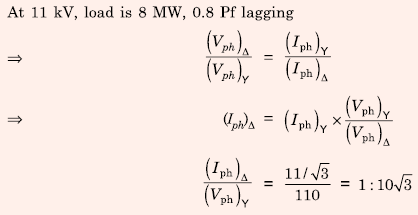Q10. Consider the function ݂f(z) = z + z* where z is a complex variable and z* denotes its complex conjugate. Which one of the following is TRUE?
Explaination / Solution: